# High School Math : Understanding Zeros of a Polynomial

## Example Questions

### Example Question #12 : Functions And Graphs

Factor the polynomial if the expression is equal to zero when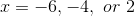.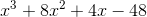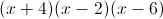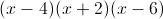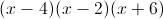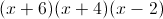Explanation:

Knowing the zeroes makes it relatively easy to factor the polynomial.

The expression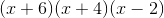fits the description of the zeroes.

Now we need to check the answer.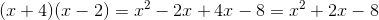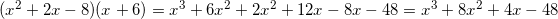We are able to get back to the original expression, meaning that the answer is.

### Example Question #13 : Pre Calculus

A polyomial with leading term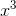has 6 as a triple root. What is this polynomial?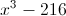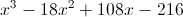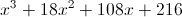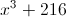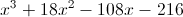Explanation:

Since 6 is a triple root, and the degree of the polynomial is 3, the polynomial is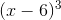, which we can expland using the cube of a binomial pattern.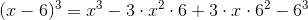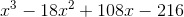### Example Question #4 : Express A Polynomial As A Product Of Linear Factors

A polyomial with leading termhas 5 and 7 as roots; 7 is a double root. What is this polynomial?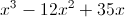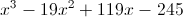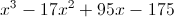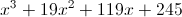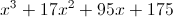Explanation:

Since 5 is a single root and 7 is a double root, and the degree of the polynomial is 3, the polynomial is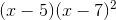. To put this in expanded form: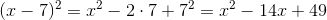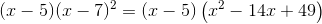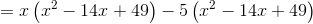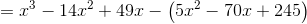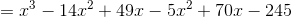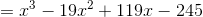### Example Question #14 : Pre Calculus

What are the solutions to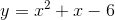?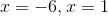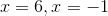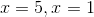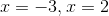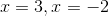Explanation:

When we are looking for the solutions of a quadratic, or the zeroes, we are looking for the values ofsuch that the output will be zero. Thus, we first factor the equation.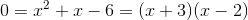Then, we are looking for the values where each of these factors are equal to zero.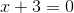implies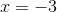and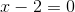impliesThus, these are our solutions.